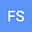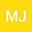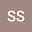Analytical solution of a fractional differential equation in the theory of viscoelastic fluids
•••• +1
• M. Javidi,
• sahar saghali,
Author ProfileM. Javidi
University of Tabriz
Author Profilesahar saghaliThe aim of this paper is to present analytical solutions of fractional delay differential equations (FDDEs) of an incompressible generalized Oldroyd-B fluid with fractional derivatives of Caputo type. Using a modification of the method of separation of variables the main equation with non-homogeneous boundary conditions is transformed into an equation with homogeneous boundary conditions, and the resulting solutions are then expressed in terms of Green functions via Laplace transforms.This results presented in two condition , in first step when $$0\leq\alpha,\beta\leq\frac{1}{2}$$ and in the second step we considered $$\frac{1}{2}\leq\alpha,\beta\leq 1$$,for each step 1,2 for the unsteady flows of a generalized Oldroyd-B fluid, including a flow with a moving plate, are considered via examples.